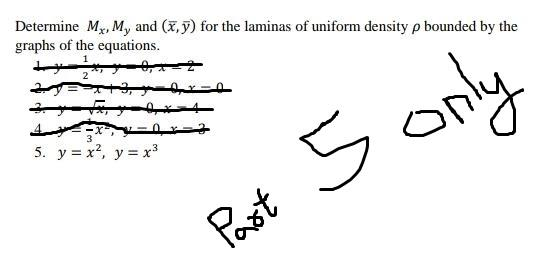### Create an Account

Already have account?

### Forgot Your Password ?

Home / Questions / Determine Mx, M, and (x,y) for the laminas of uniform density p bounded by the graphs of t...

# Determine Mx, M, and (x,y) for the laminas of uniform density p bounded by the graphs of the equations. 1 5. y = x?, y = x3 5 only Past

Determine Mx, M, and (x,y) for the laminas of uniform density p bounded by the graphs of the equations. 1 5. y = x?, y = x3 5 only PastApr 13 2021 View more View Less

#### Answer (Solved)Subscribe To Get Solution